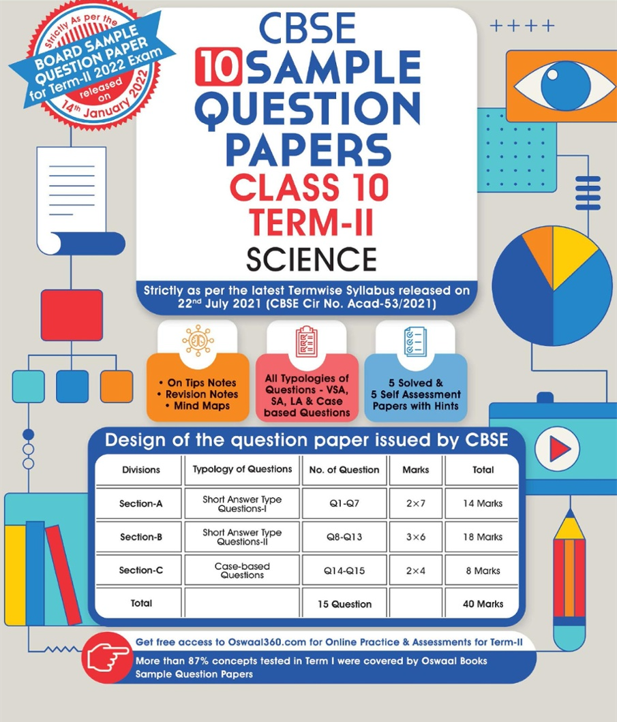# Calculate the total resistance of the circuit and find the total current in the circuit.

CBSE Sample Question Paper, Class 10 Science Term 2 Question 1 - (Calculate the total resistance of the circuit and find the total current in the circuit.)